# 单位圆"单位圆" 是半径为 1 的圆。## 正弦、余弦和正切cos 0° = 1、sin 0° = 0、tan 0° = 0cos 90° = 0、sin 90° = 1、tan 90°是未定义

## 自己来试试！## 勾股

x2 + y2 = 12

x2 + y2 = 1

(cos(θ))2 + (sin(θ))2 = 1

## 重要的角：30°、45° 和 60°

30°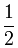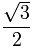1 √3 = √3 3
45°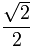1
60°√3

### 怎样去记？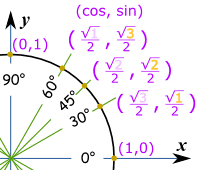sin(30°)  = √1 = 1 (because √1 = 1) 2 2
 sin(45°)  = √2 2
 sin(60°)  = √3 2

 cos(30°)  = √3 2
 cos(45°)  = √2 2
 cos(60°)  = √1 = 1 (because √1 = 1) 2 2

## 只要记住三个数

 只要记住三个数就够了： 1 , √2 and √3 2 2 2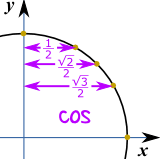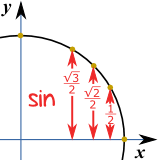## 正切呢？

 tan(30°) = sin(30°) = 1/2 = 1 cos(30°) √3/2 √3
但写 1/√3 可能会扣分
（见有理分母），所以应该这样写：√3/3
 tan(45°) = sin(45°) = √2/2 = 1 cos(45°) √2/2

 tan(60°) = sin(60°) = √3/2 = √3 cos(60°) 1/2

## 画个草图

 画一个边长为 2的三角形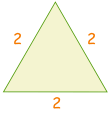把三角形一分为二。勾股定理说新的边的长度是 √3 12 + (√3)2 = 22 1 + 3 = 4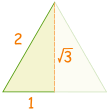然后用正弦、余弦和正切的定义来记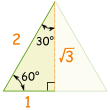### 例子：sin(30°)

sin(30°) = 对边 斜边 = 1 2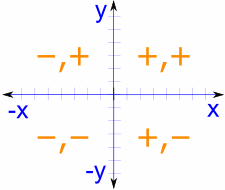## 全圆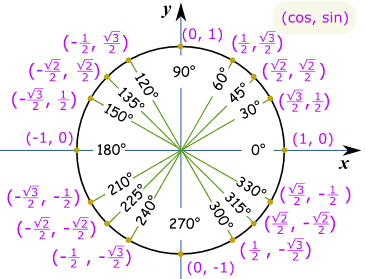### 例子：cos(330°) 是多少？?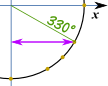画个草图，我们可以看到答案是 "长" 的值： √3 2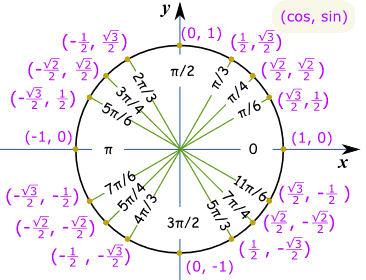### 例子：sin(7π/6) 是多少？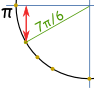### 脚注：这些值是怎样计算出来的？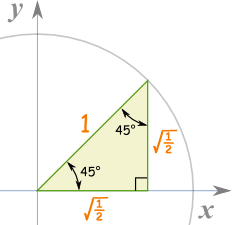### 45度

45度：x 与 y 相等，所以 y=x

x2 + x2 = 1
2x2 = 1
x2 = ½
x = y = √(½)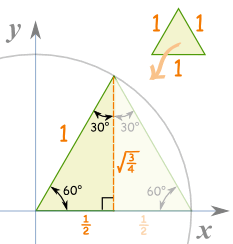"x" 边变成 ½,

"y" 边是：

(½)2 + y2 = 1
¼ + y2 = 1
y2 = 1-¼ = ¾
y = √(¾)

### 30度

30°就是 x 和 y 对调了的 60°，所以 x = √(¾)y = ½

 √(½) 也等于：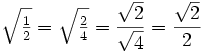√(¾) 也等于：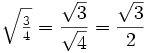30°1 √3 = √3 3
45°1
60°√3